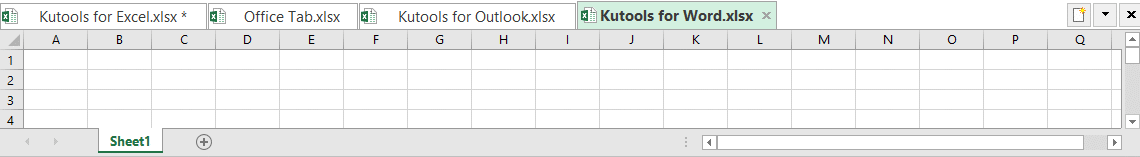## How to sum top 3 or n values based on criteria in Excel?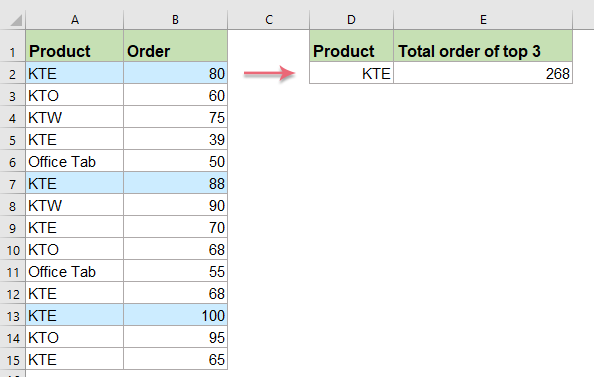Normally, we can sum the top n values from a range of cells by using a simple formula, but have you ever tried to sum top n values based on some criteria. This article, I will introduce some formulas for summing top n values with specific criteria in Excel.

Sum top 3 or n values based on one condition with formulas

Sum top 3 or n values based on multiple criteria with formulas

#### Sum top 3 or n values based on one condition with formulas

To sum the top 3 or n values with one condition, you can apply the below formulas:

The generic formula is:

=SUMPRODUCT(LARGE((range=criteria)*(values),{1,2,3,N}))
• range=criteria is the range of cells that matches with specific criteria.
• values is the list of numbers values which contain the top values to be summed.
• N represents the Nth top value.

1. Please apply this formula into a blank cell:

=SUMPRODUCT(LARGE((\$A\$2:\$A\$15=D2)*(\$B\$2:\$B\$15),{1,2,3}))
• Tips: In this formula:
• \$A\$2:\$A\$15=D2: is the range that matches the specific criteria.
• \$B\$2:\$B\$15: is the list of values which contain the top values you want to sum.
• 1,2,3: indicates the top 3 values you want to sum.

2. Then, press Enter key to get the result of the total values of the top 3 values, see screenshot: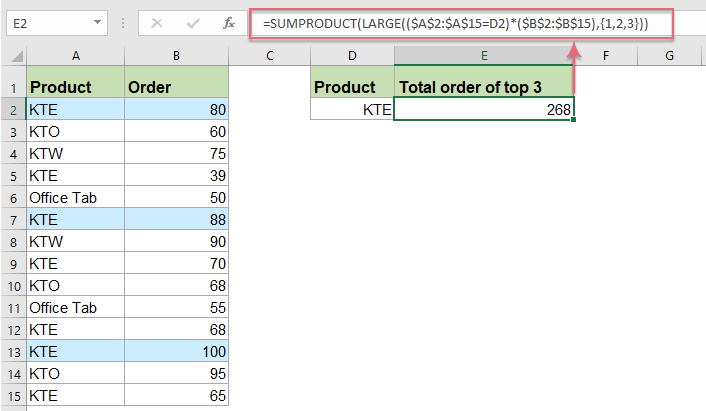Note: Accept the above formula, you can also use an array formula to deal with this job:

=SUM(IFERROR(LARGE(IF((\$A\$2:\$A\$15=D2),\$B\$2:\$B\$15),{1,2,3}),0))

Remember to press Ctrl + Shift + Enter key together to get the correct result.

#### Sum top 3 or n values based on multiple criteria with formulas

If you need to sum the top n values based on two or more criteria, for example, I want to sum the top 3 orders of product KTE that salesman is Kerry as following screenshot shown: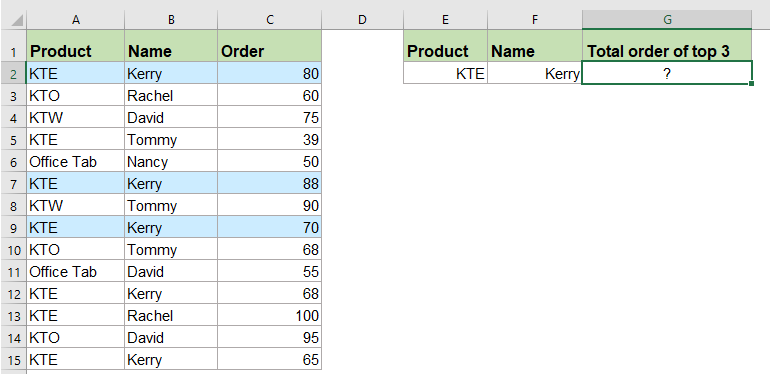To solve this task, the generic formula is:

=SUMPRODUCT(LARGE((range1=criteria1)*(range2=criteria2)*(values),{1,2,3,N}))
• range1=criteria1 is the first range of cells that matches with specific criteria1.
• range2=criteria2 is the second range of cells that matches with specific criteria2.
• values is the list of numbers values which contain the top values to be summed.
• N represents the Nth top value.

1. Please apply the below formula into a blank cell:

=SUMPRODUCT(LARGE((\$A\$2:\$A\$15=E2)*( \$B\$2:\$B\$15=F2)*(\$C\$2:\$C\$15),{1,2,3}))

2. And then, press Enter key, you will get the result as you need: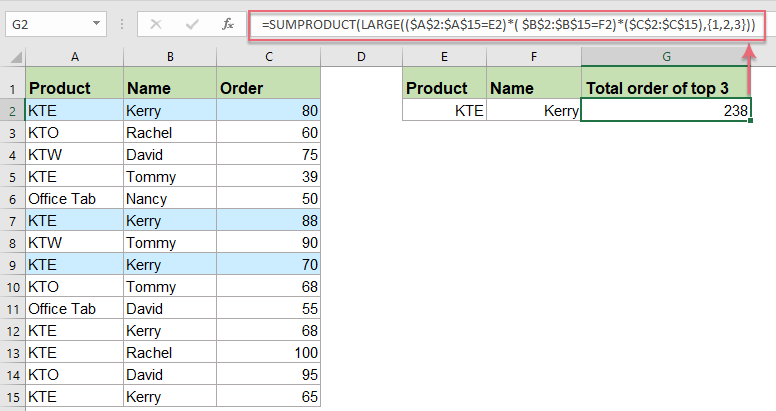Note: You can also use an array formula to solve this job:

=SUM(IFERROR(LARGE(IF((\$A\$2:\$A\$15=E2)*( \$B\$2:\$B\$15=F2), \$C\$2:\$C\$15),{1,2,3}),0))

Please remember to press Ctrl + Shift + Enter key together to get the correct result.

#### More relative sumif values articles:

• Sumif With Multiple Criteria In One Column
• In Excel, SUMIF function is a useful function for us to sum cells with multiple criteria in different columns, but with this function, we can also sum cells based on multiple criteria in one column. In this article. I will talk about how to sum values with more than one criteria in the same column.
• Sumif With One Or More Criteria In Excel
• In Excel, sum values based on one or more criteria is a common task for most of us, the SUMIF function can help us to quickly sum the values based on one condition and the SUMIFS function help us to sum values with multiple criteria. This article, I will describe how to sum with one or more criteria in Excel?
• Sumif Adjacent Cell Is Equal, Blank Or Containing Text In Excel
• While using Microsoft Excel, you may need to sum values where the adjacent cell equals a criterion in a certain range, or sum the values where the adjacent cell is blank or containing text. In this tutorial, we will provide you with formulas to deal with these problems.
• Sumif Based On Partial Match In Excel
• Take the below data as an example, I want to sum cell values in column B when there contains partial “KTE” text in column A. How to achieve it? This article, I will talk about how to sum cell values based on a partial match in Excel worksheet.
• Sumif Cell Values Between Two Given Dates In Google Sheets
• In my Google sheet, I have two columns which contain a date column and order column, now, I want to sum the order column cells based on the date column. For example, sum values between 2018/5/15 and 2018/5/22 as following screenshot shown. How could you solve this job in Google sheets?

## The Best Office Productivity Tools

### Kutools for Excel Solves Most of Your Problems, and Increases Your Productivity by 80%

• Super Formula Bar (easily edit multiple lines of text and formula); Reading Layout (easily read and edit large numbers of cells); Paste to Filtered Range...
• Merge Cells/Rows/Columns and Keeping Data; Split Cells Content; Combine Duplicate Rows and Sum/Average... Prevent Duplicate Cells; Compare Ranges...
• Select Duplicate or Unique Rows; Select Blank Rows (all cells are empty); Super Find and Fuzzy Find in Many Workbooks; Random Select...
• Exact Copy Multiple Cells without changing formula reference; Auto Create References to Multiple Sheets; Insert Bullets, Check Boxes and more...
• Favorite and Quickly Insert Formulas, Ranges, Charts and Pictures; Encrypt Cells with password; Create Mailing List and send emails...
• Extract Text, Add Text, Remove by Position, Remove Space; Create and Print Paging Subtotals; Convert Between Cells Content and Comments...
• Super Filter (save and apply filter schemes to other sheets); Advanced Sort by month/week/day, frequency and more; Special Filter by bold, italic...
• Combine Workbooks and WorkSheets; Merge Tables based on key columns; Split Data into Multiple Sheets; Batch Convert xls, xlsx and PDF...
• Pivot Table Grouping by week number, day of week and more... Show Unlocked, Locked Cells by different colors; Highlight Cells That Have Formula/Name...### Office Tab - brings tabbed interface to Office, and make your work much easier

• Enable tabbed editing and reading in Word, Excel, PowerPoint, Publisher, Access, Visio and Project.
• Open and create multiple documents in new tabs of the same window, rather than in new windows.
• Increases your productivity by 50%, and reduces hundreds of mouse clicks for you every day!